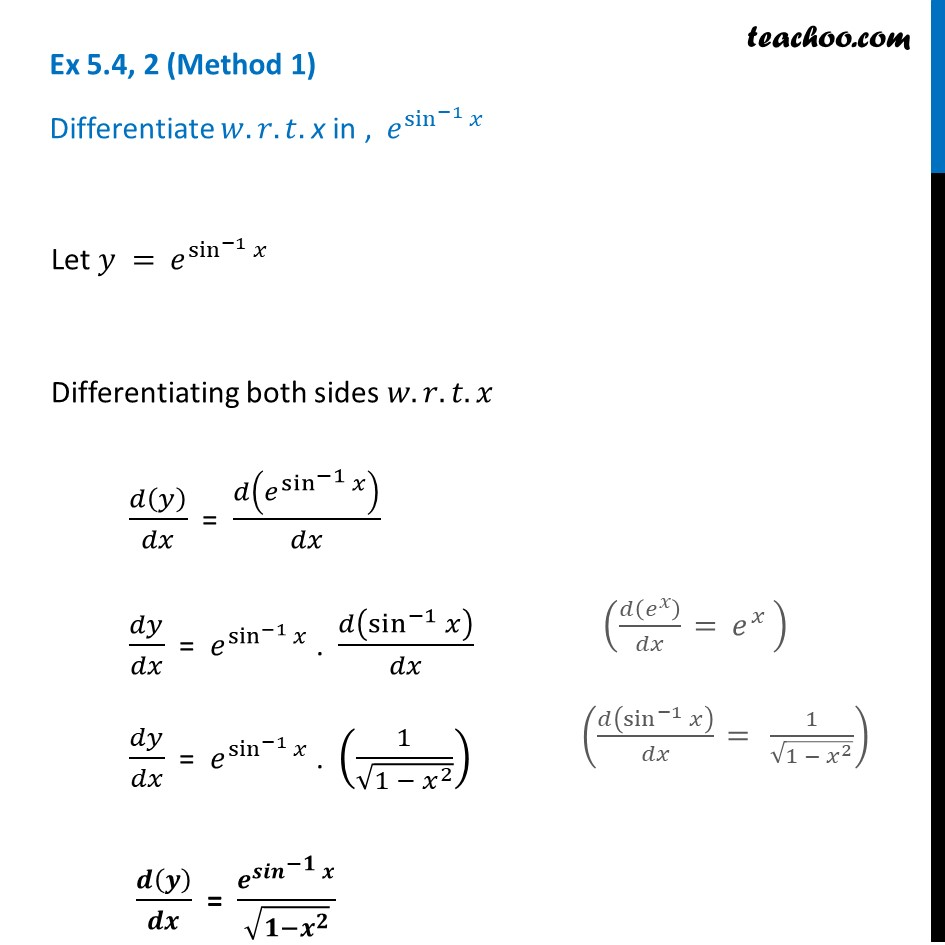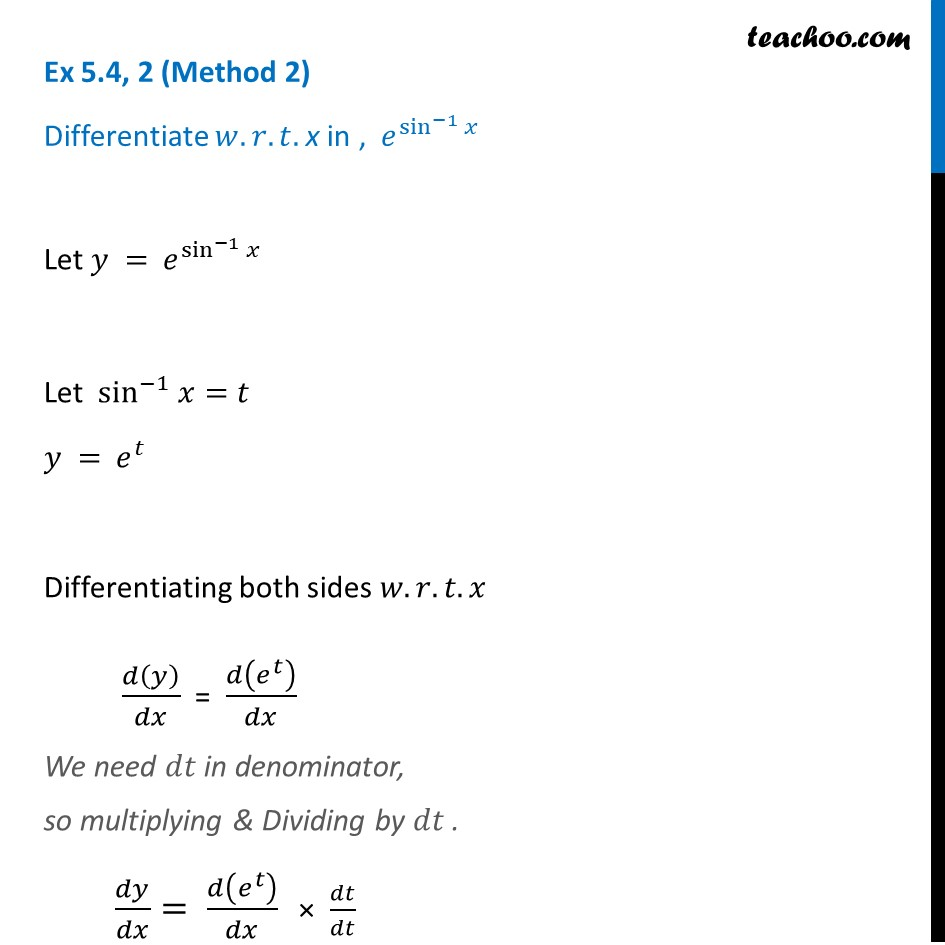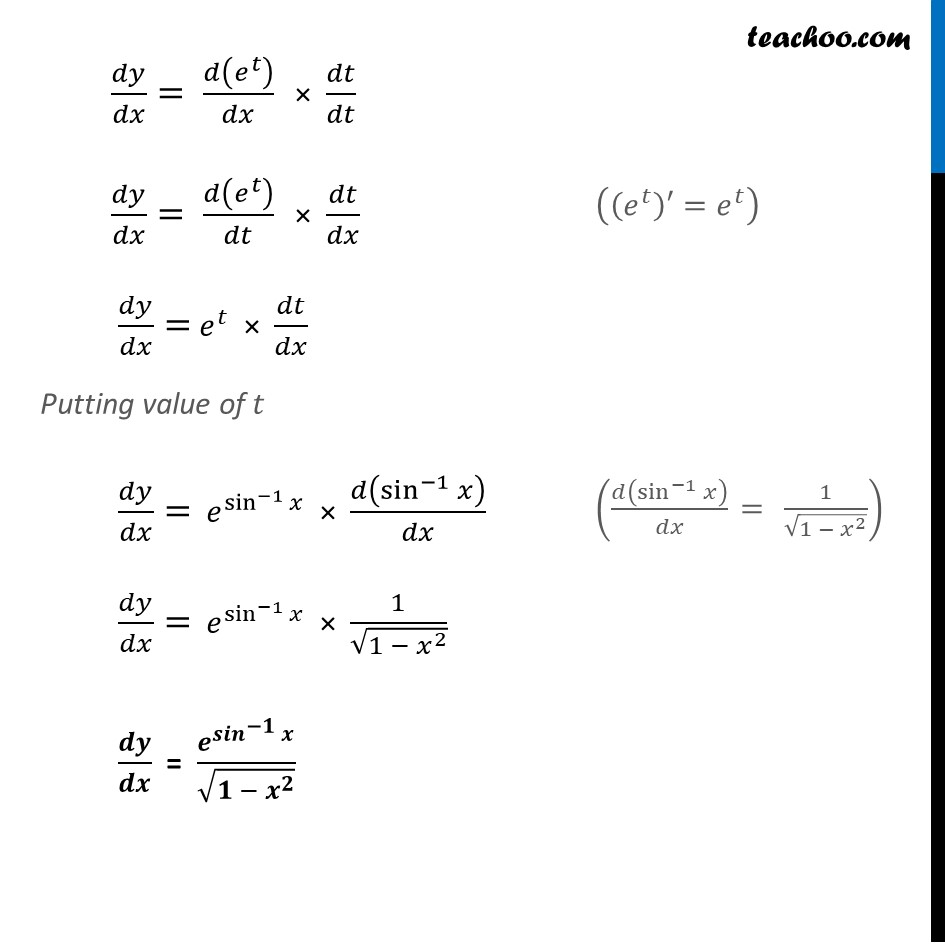Finding derivative of Exponential & logarithm functions

Chapter 5 Class 12 Continuity and Differentiability
Concept wiseIntroducing your new favourite teacher - Teachoo Black, at only ₹83 per month

### Transcript

Ex 5.4, 2 (Method 1) Differentiate 𝑤.𝑟.𝑡. x in , 𝑒^(sin^(−1) 𝑥)Let 𝑦 = 𝑒^(sin^(−1) 𝑥) Differentiating both sides 𝑤.𝑟.𝑡.𝑥 𝑑(𝑦)/𝑑𝑥 = 𝑑(𝑒^(sin^(−1) 𝑥) )/𝑑𝑥 𝑑𝑦/𝑑𝑥 = 𝑒^(sin^(−1) 𝑥) . 𝑑(sin^(−1) 𝑥)/𝑑𝑥 𝑑𝑦/𝑑𝑥 = 𝑒^(sin^(−1) 𝑥) . (1/√(1 − 𝑥^2 )) 𝒅(𝒚)/𝒅𝒙 = 𝒆^(〖𝒔𝒊𝒏〗^(−𝟏) 𝒙)/√(𝟏−𝒙^𝟐 ) (𝑑(𝑒^𝑥 )/𝑑𝑥 " = " 𝑒^𝑥 " " ) Ex 5.4, 2 (Method 2) Differentiate 𝑤.𝑟.𝑡. x in , 𝑒^(sin^(−1) 𝑥)Let 𝑦 = 𝑒^(sin^(−1) 𝑥) Let sin^(−1) 𝑥=𝑡 𝑦 = 𝑒^𝑡 Differentiating both sides 𝑤.𝑟.𝑡.𝑥 𝑑(𝑦)/𝑑𝑥 = 𝑑(𝑒^𝑡 )/𝑑𝑥 We need 𝑑𝑡 in denominator, so multiplying & Dividing by 𝑑𝑡 . 𝑑𝑦/𝑑𝑥= 𝑑(𝑒^𝑡 )/𝑑𝑥 × 𝑑𝑡/𝑑𝑡 𝑑𝑦/𝑑𝑥= 𝑑(𝑒^𝑡 )/𝑑𝑥 × 𝑑𝑡/𝑑𝑡 𝑑𝑦/𝑑𝑥= 𝑑(𝑒^𝑡 )/𝑑𝑡 × 𝑑𝑡/𝑑𝑥 𝑑𝑦/𝑑𝑥= 𝑒^𝑡 × 𝑑𝑡/𝑑𝑥 Putting value of 𝑡 𝑑𝑦/𝑑𝑥= 𝑒^(sin^(−1) 𝑥) × 𝑑(sin^(−1) 𝑥)/𝑑𝑥 𝑑𝑦/𝑑𝑥= 𝑒^(sin^(−1) 𝑥) × 1/√(1 − 𝑥^2 ) 𝒅𝒚/𝒅𝒙 = 𝒆^(〖𝒔𝒊𝒏〗^(−𝟏) 𝒙)/√(𝟏 − 𝒙^𝟐 )